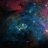# How do I convert watts to amps?

Converting watts to amps is a fundamental concept in electrical engineering. In this article, we will explore the process of converting watts to amps, the relationship between these units, and practical considerations when making conversions. The article is organized into the following sections:

## Understanding the Basics​

### Ohm's Law and Power​

To understand how to convert watts to amps, it is essential to first understand Ohm's Law and the power equation. Ohm's Law states that voltage (V) is equal to current (I) multiplied by resistance (R):

V = I × R

The power equation, on the other hand, relates power (P) to voltage (V) and current (I):

P = V × I

### Relationship between watts and amps​

Watts (W) are a unit of electrical power, while amps (A) are a unit of electrical current. By combining the power equation with Ohm's Law, we can derive a formula to convert watts to amps, given either voltage or resistance.

## Conversion Scenarios and Calculations​

### Converting watts to amps with known voltage​

If you know the voltage (V) and power (P) of a circuit, you can calculate the current (I) using the formula:

I = P / V

For example, if you have a 100-watt light bulb running on a 120-volt circuit, the current can be calculated as:

I = 100 W / 120 V ≈ 0.83 A

### Converting watts to amps with known resistance​

If you know the resistance (R) and power (P) of a circuit, you can calculate the current (I) using the formula:

I = √(P / R)

For example, if a 100-watt heating element has a resistance of 50 ohms, the current can be calculated as:

I = √(100 W / 50 Ω) ≈ 0.45 A

## Safety Considerations and Tips​

When converting watts to amps, always keep the following safety tips in mind:

1. Ensure the voltage and resistance values are accurate for the specific device or circuit you are working with.
2. Be aware of the maximum current rating of your circuit components, as exceeding this rating can lead to overheating and potential failure.
3. Always use a multimeter to verify the actual current in your circuit, as calculations are based on ideal conditions and may not always reflect real-world behavior.

## Conclusion​

Converting watts to amps is an essential skill for anyone working with electrical systems. By understanding Ohm's Law and the power equation, you can easily calculate the current in a circuit when given either voltage or resistance values. Always remember to prioritize safety and verify your calculations with actual measurements to ensure the proper functioning of your electrical devices and systems.
Author
logic
Views
709
First release
Last update
Rating
1 ratings

#### More resources from logic